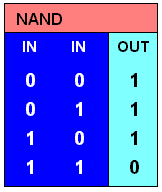# truth table

Also found in: Dictionary, Wikipedia.

## truth table

[′trüth ‚tā·bəl]
(mathematics)
A table listing statements concerning an event and their respective truth values.

## truth table

(logic)
A table listing all possible combinations of inputs and the corresponding output of a Boolean function such as AND, OR, NOT, IMPLIES, XOR, NAND, NOR. Truth tables can be used as a means of representing a function or as an aid in designing a circuit to implement it.

## truth table

A chart of a logical operation's inputs and outputs. The following example is a Boolean NAND truth table.References in periodicals archive ?
In addition to generating a truth table, the researchers checked the consistency of the data over extended run times to help identify error sources in the experimental setup.
Next, a truth table algorithm for fuzzyset QCA (fs/QCA) was set up to summarize the data synthetically.
Keywords: system controlled by computer, dependability, optimization qualitative and quantitative, truth table, Karnaugh table, reduced Markov graph
The result of the probability connector between the two fuzzy propositions (A) and (B) in the following truth table:
Figure 1: Schematic diagram and truth table for AND operation based on SOA-MZI.
The 2P-1N three-transistor structure  and C-element circuit  and their truth tables are shown in Figure 4.
Applying Boolean algebra to the matrix, coding all values >0.5 as 1, and those <0.5 as 0, we created a truth table indicating the various combinations of conditions among hospitals ranked by their underuse rates.
Table I: various operations using basic GDI cell N p G out operation '0' y X [bar.x]y F1 y '1' X [bar.x] F2 4- y '1' y X x+y OR y '0' X xy AND z y X [bar.x]y MUX + xz '0' '1' X [bar.x] NOT Table II: Truth Table Of Full Adder Inputs Outputs A B Cin S Cout 0 0 0 0 0 0 0 1 1 0 0 1 0 1 0 0 1 1 0 1 1 0 0 1 0 1 0 1 0 1 1 1 0 0 1 1 1 1 1 1 Table III: Comparison of GDI and Conventional Full adder Number of XOR AND OR Total No.
Those familiar with Simulink[R] may ask "Why not use the built-in truth table function instead of manually constructing a TT from transitions and junction blocks?" To illustrate, the figure below shows the TT implemented using the built-in Truth Table Object (TTO) in Simulink/Stateflow[R]:
By modifying the inputs to make its truth table corresponding to AND and NOT gates, one can easily show that the Toffoli gate is universal.
To count pass and fail cases for truth table (Table 1), following method was adopted: if a corner detector detects a corner pixel in an original image, it was matched against three values in the validation image.

Site: Follow: Share:
Open / Close